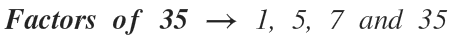Select Page

# Factors of 35

The number 35 has 4 factors and is a composite number.

1. To calculate the factors of 35 we divide, whenever we can, among all the numbers that are less than it.

35:1=35
35:5=7
35:7=5
35:35=1